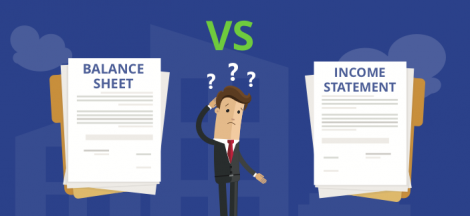Probability

Business decisions are mostly based on an analysis of uncertainties such as the following;

1. What are the “chances” that sales will decline if we increase prices of goods?

2. What is the “likelihood” that a new assembly method would boost productivity?

3. How “likely” is it that the project to be completed on time?

4. What are the “chances” of a new investment being profitable?

Probability is a numeric measure that is used to determine the likelihood that an event will occur. Many events cannot be predicted with total certainty. We can predict only the chance of an event to occur i.e., how likely they are going to happen, using it.

Thus, probabilities could be used as measures of the degree of uncertainty associated with the four events previously listed. If probabilities were available, we could determine the likelihood of each event occurring.

Probability values are always assigned on a 0 to 1 scale. A probability of 0 implies that an event is unlikely to occur (is an impossible event); a probability of 1 suggests that an event is almost certain to occur (is a certain event). Other probabilities ranging from 0 to 1 represent varying degrees of certainty that an event will occur. The lower the likelihood, the less likely the related event will occur; the higher the probability, the more likely the connected event will occur.

Tossing a fair (unbiased) coin is a basic example. Because the coin is fair, the two outcomes (“heads” and “tails”) are equally likely; the chance of “heads” equals the probability of “tails,” and because no other outcomes are possible, the probability of either “heads” or “tails” is 1/2. (Which could also be written as 0.5 or 50 percent).

Probability is crucial in decision making because it allows us to quantify, articulate, and understand the uncertainties that accompany future events.

When considering probability, we define an experiment as any process that produces well-defined results. One and only one of the possible experimental outcomes will occur on each one repetition of an experiment.

Terminology of Probability Theory

The probability terms listed below can help one better understand some probability concepts.

Experiment: An experiment is a trial or operation that is carried out to produce a result.

A sample space is made up of all the possible outcomes of an experiment. Tossing a coin, for example, has a sample space of head and tail.

Favorable Outcome: An event that has produced the desired result or expected event is called a favorable outcome. For example, when we roll two dice, the possible/favorable outcomes of getting the sum of numbers on the two dice as 4 are (1,3), (2,2), and (3,1).

A trial is the act of conducting a random experiment.

Random Experiment: A random experiment is one that has a well-defined set of outcomes. For example, when we toss a coin, we know whether we will get heads or tails, but we don’t know which one.

An event is the total number of outcomes of a random experiment.## Understanding the Balance Sheet and the Income Statement: A Guide for Business Managers #MMBA5

in